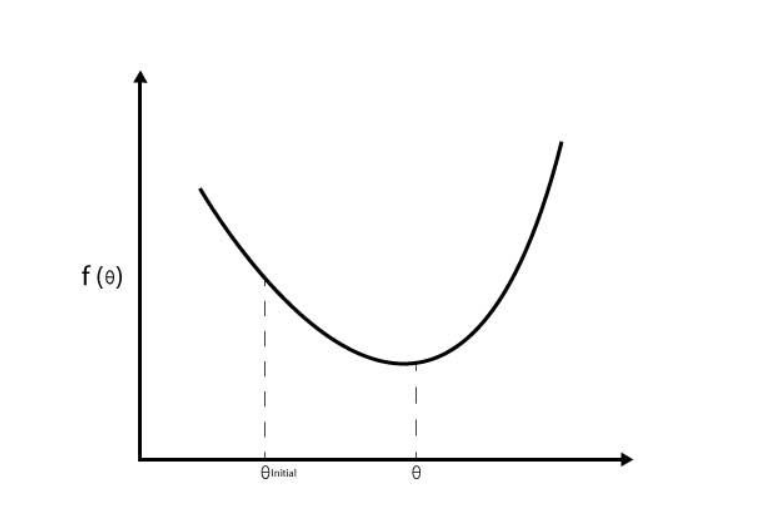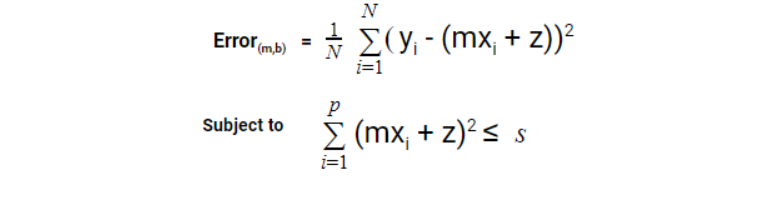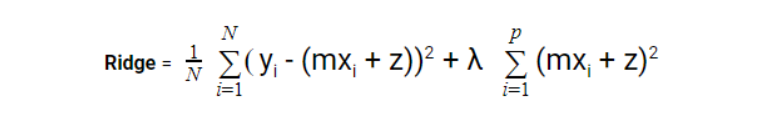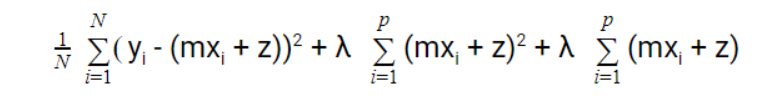• Category
• >Machine Learning

# Linear, Lasso, Ridge, and Elastic Net Regression: An Overview

• Tanesh Balodi
• Jul 16, 2020
• Updated on: Feb 09, 2021It might not be a good start, to begin with, Ridge, elastic and Quantile Regression, assuming that not many are aware of the Regression topic, therefore, here we will discuss a little bit about Linear regression in order to give quite a basic but effective approach towards our main topic.

## What is Regression?

Regression is one of the most popular and probably the easiest machine learning algorithms. It comes under supervised learning (label specified problems).

Regression basically helps out in finding the relation between independent variables and dependent variables.

So what are dependent and independent variables?

Dependent variables represent a quantity whose value depends upon how the independent variables are changed. Also defined as the effect.

whereas independent variables represent a quantity that is being changed and is independent of others variables. Also defined as the cause.Graph between Plant Height( y-axis) and Time(x-axis)

In the above example, plant height depends upon the time(days) which represents that plant height(y-axis) is the dependent variable and on the other hand, time(x-axis) is the independent variable.

The relation between the dependent and independent variables is shown with the help of the linear line, often known as the best split line or decision boundary.

## Decision Boundary

Decision boundary is a hypersurface that separates the underlying vector space into sets depending on the number of classes. Decision boundaries can be linear (in logistic regression) and also can be non-linear (like in random forest classifier).Graph between Predicted Value(y-axis) and Actual Value(x-axis)

## Steps for Making the Best Decision Boundary:

1. ### Linear Regression Formula -:

Yi = MXi + Z

Here, Yi represents the dependent variable, M is slope complexity, Xi is an independent variable, and Z is the intercept also known as Bias. This is nothing but a Linear line equation which is also known as the hypothesis equation in machine learning. Here Yi is predicted output and Xi is input or actual value.

Initially, the variables are scattered, to obtain the best fit line we will calculate the error.

1. ### Calculation of Error:

Initially, the model will predict a line with a huge error, In order to train the model, we must calculate the error. Error is nothing but the difference between predicted and actual value.

The equation for calculation of error is -:Graph of the difference between predicted value(y-axis) and the actual value(x-axis)

Above is the error function of the regression model, Yi is the actual vertical distance, and (mxi + b) is the hypothesis equation. We have squared the error in order to get positive values and divided by N in order to ease the computation.The graph between the square of the error between the predicted value and actual value

Once we have calculated the error, we desire to minimize the error so that we can conclude the best split line. We have a method known as Gradient Descent for minimization of error. Also, this gradient descent helps in the optimization of convex functions(which have only one local minimum).Graphical Representation of Convex function

#### PSEUDO CODE:

1. Random value of initial weight

2. Measure how good the weight is -> error function

3. Minimization of error by gradient descent

After using all three steps we will check the problem like overfitting as well as underfitting which generally occurs in linear regression;

#### Overfitting and Underfitting

• Overfitting is a condition where bias is low but variance tends to get high and results in fitting too much in a model, whereas in
• Underfitting, the variance is low but bias tends to get high and the model becomes too loose or simplified.

Also, when we can’t examine anything from the machine learning algorithm, we can say it is an underfitting condition, whereas, when data provides excessive information that we don’t even need and this data is acting as a burden, we say it is an overfitting problem.Graphical representation of Underfitting, Just Fitting, and Overfitting

So, how to prevent these problems in regression? The solution is Regression models.

## What is Ridge Regression?

Ridge regression is a method to perform linear regression with fewer chances of a model getting into problems such as underfitting or overfitting.

• It is used highly for the treatment of multicollinearity in regression, it means when an independent variable is correlated in such a way that both resemble each other,

• It causes high variance among the independent variables, we can change the value of the independent variable but it will cause a loss of information.A view of the variation of variance and bias in Ridge Regression

So in Ridge regression, we make bias and variance proportional to each other, or it basically decreases the difference between actual and predictive values.

It is known to be one of the techniques used to set up a parsimonious model, i.e a model that uses fewer predictors but fully achieves its goals.

The normal linear regression equation for error is -:For ridge regression, Error function remains the same i.e.;Where ‘s’ is constrained value, it penalizes the bigger coefficient and therefore manages to shrink the biases accordingly in order to make proportionate with variance. This regularization is also known as L2 regularization.To conclude together, the equation looks like this, here λ is a value that controls the level or shrinking of biases and can never be zero or less than zero.

## What is Lasso Regression?

Lasso stands for Least Absolute Shrinkage Selector Operator

• It works the same as ridge regression when it comes to assigning the penalty for coefficient,

• It removes the coefficient and the variables with the help of this process and limits the bias through the below formulaIt is known as L1 Regularization.

## What is Elastic Net Regression?

Coefficient to the variables are considered to be information that must be relevant, however, ridge regression does not promise to remove all irrelevant coefficient which is one of its disadvantages over Elastic Net Regression(ENR)

It uses both Lasso as well as Ridge Regression regularization in order to remove all unnecessary coefficients but not the informative ones.

ENR = Lasso Regression + Ridge Regression

The equation for ENR is given below-:“The energy of youth with the experience of age is a lethal combination.” ― Murad S. Shah

Justified! , we just saw the power of combination.

## Difference between Ridge, Lasso and Elastic Net Regression

1. In terms of handling bias, Elastic Net is considered better than Ridge and Lasso regression, Small bias leads to the disturbance of prediction as it is dependent on a variable. Therefore Elastic Net is better in handling collinearity than the combined ridge and lasso regression.

1. Also, When it comes to complexity, again, Elastic Net performs better than ridge and lasso regression as both ridge and lasso, the number of variables is not significantly reduced. Here, incapability of reducing variables causes declination in model accuracy.

1. Ridge and Elastic Net could be considered better than the Lasso Regression as Lasso regression predictors do not perform as accurately as Ridge and Elastic Net. Lasso Regression tends to pick non-zero as predictors and sometimes it affects accuracy when relevant predictors are considered as non zero.

## Conclusion

Undoubtedly, regression is a widely used technique, we just read about the Ridge, Lasso, and Elastic net Regression and how they help in regularization.

Regression is an interesting topic for every Machine Learning enthusiast and fundamentals of machine learning also. For more blogs in Analytics and new technologies do read Analytics Steps.

•#### 96sudeshnasen

Jul 02, 2022

Good summary. But why is the regularization parameter in the loss function given as mx+z instead of just the m (or slopes)? In the difference section towards the end, "Elastic Net is better in handling collinearity than the combined ridge and lasso regression." what does this mean? Isn't the combination of Ridge & Lasso itself called Elastic Net?

•#### maneeeshak443

Mar 16, 2023

I didn’t think that the content of this post would be so rich that I will end up sharing the link with so many people when I first saw this online. With every single line, the writer has managed to unravel some interesting concepts about data science that I never knew existed. This post motivated me to read more on this subject, and I am forever thankful to the writer for introducing me to a new world through this post. <a href="https://360digitmg.com/india/hyderabad/data-science-certification-course-training-institute">best data science course in hyderabad with placement</a>

•